Showing first {{hits.length}} results of {{hits_total}} for {{searchQueryText}}{{hits.length}} results for {{searchQueryText}}

Ingen søkeresultater

LaTeX typesetting is made by using special tags or commands that provide a handful of ways to format your document. Sometimes standard commands are not enough to fulfil some specific needs, in such cases new commands can be defined and this article explains how. Contents 1 Introduction 2 Commands 3 Defining a new command 3.1 Simple commands 3.2 Commands with parameters 3.3 Commands with optional parameters 4 Overwriting existing commands 5 Further reading Introduction Most of the LaTeX commands are simple words preceded by a special character. In a document there are different types of \textbf{commands} that define the way the elements are displayed. This commands may insert special elements: $\alpha \beta \Gamma$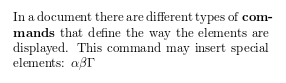In the previous example there are different types of commands. For instance, \textbf will make boldface the text passed as parameter to the command. In mathematical mode there are special commands to display Greek characters.   Open an example in Overleaf Commands Commands are special words that determine LaTeX behaviour. Usually this words are preceded by a backslash and may take some parameters. Example a list \begin{itemize} \item[\S] First item \item Second item \end{itemize}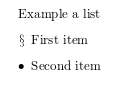The command \begin{itemize} starts an environment, see the article about environments for a better description. Below the environment declaration is the command \item, this tells LaTeX that this is an item part of a list, and thus has to be formatted accordingly, in this case by adding a special mark (a small black dot called bullet) and indenting it. Some commands need one or more parameters to work. The example at the introduction includes a command to which a parameter has to be passed, textbf; this parameter is written inside braces and it's necessary for the command to do something. There are also optional parameters that can be passed to a command to change its behaviour, this optional parameters have to be put inside brackets. In the example above, the command \item[\S] does the same as item, except that inside the brackets is \S that changes the black dot before the line for a special character.   Open an example in Overleaf Defining a new command LaTeX is shipped with a huge amount of commands for a large number of tasks, nevertheless sometimes is necessary to define some special commands to simplify repetitive and/or complex formatting. Simple commands New commands are defined by \newcommand statement, let's see an example of the simplest usage. \newcommand{\R}{\mathbb{R}} The set of real numbers are usually represented by a blackboard bold capital r: $$\R$$.The statement \newcommand{\R}{\mathbb{R}} has two parameters that define a new command \R This is the name of the new command. \mathbb{R} This is what the new command does. In this case the letter R will be written in blackboard boldface style. (to use \mathbb the package amssymb is needed) After the command definition you can see how the command is used in the text. Even tough in this example the new command is defined right before the paragraph where it's used, good practice is to put all your user-defined commands in the preamble of your document.   Open an example in Overleaf Commands with parameters It is also possible to create new commands that accept some parameters. \newcommand{\bb}{\mathbb{#1}} Other numerical systems have similar notations. The complex numbers $$\bb{C}$$, the rational numbers $$\bb{Q}$$ and the integer numbers $$\bb{Z}$$.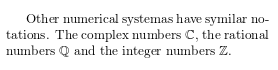The line \newcommand{\bb}{\mathbb{#1}} defines a new command that takes one parameter. \bb This is the name of the new command.  The number of parameters the new command will take. \mathbb{#1} This is what the command actually does. In this case the parameter, referenced as $1 , w i l l b e w r i \texttt{e} n u \sin g b l a c k b o a r d b o l \mathrm{df} a c e c h a r a c t e r s . I f t h e \mathrm{de} f \in e d \ne w c o m m \mathmr{and} \ne e \mathrm{ds} m \mathmr{and} e t h a n o \ne p a r a m e t e r , y o u c a n r e f e r e a c h p a r a m e t e r b y$1, #2 and so on, up to 9 parameters are supported.   Open an example in Overleaf Commands with optional parameters User-defined commands are even more flexible than the examples shown above. You can define commands that take optional parameters: \newcommand{\plusbinomial}{($2 +$3)^#1} To save some time when writing too many expressions with exponents is by defining a new command to make simpler: $\plusbinomial{x}{y}$ And even the exponent can be changed $\plusbinomial{y}{y}$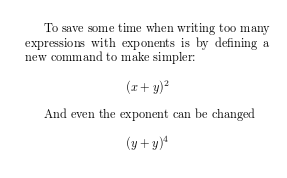Let's analyse the syntax of the line \newcommand{\plusbinomial}{($2 +$3)^#1}: \plusbinomial This is the name of the new command.  The number of parameters the command will take, in this case 3.  Is the default value for the first parameter. This is what makes the first parameter optional, if not passed it will use this default value. ($2 +$3)^#1 This is what the command does. In this case it will put the second and third parameters in a "binomial format" to the power represented by the first parameter.   Open an example in Overleaf Overwriting existing commands If you define a command that has the same name as an already existing LaTeX command you will see an error message in the compilation of your document and the command you defined will not work. If you really want to override an existing command this can be accomplished by \renewcommand: \renewcommand{\S}{\mathbb{S}} The Riemann sphere (the complex numbers plus $\infty$) is sometimes represented by $$\S$$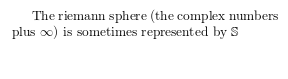In this example the command \S (see the example in the commands section) is overwritten to print a blackboard bold S. \renewcommand uses the same syntax as \newcommand.   Open an example in Overleaf Further reading For more information see: Environments Understanding packages and class files Writing your own package Writing your own class Lengths in LaTeX Using colours in LaTeX Page size and margins List of packages and class files The not so short introduction to LaTeX2ε LaTeX/Creating_Packages on WikiBooks